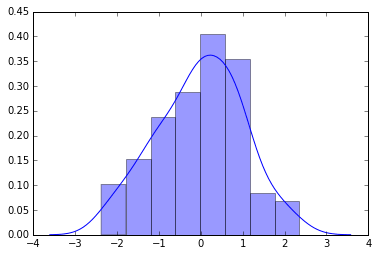Seaborn by Example: Data Visualization and Plotting using Python

# Seaborn by Example: Data Visualization and Plotting using Python

Last updated:

WIP Alert This is a work in progress. Current information is correct but more content may be added in the future.

## Seaborn vs Matplotlib

Seaborn is not a replacement for Matplotlib.

• Seaborn is a higher-level interface to Matplotlib.

• It uses Matplotlib behind the scenes.
• Seaborn has much tighter integration with Pandas.

• For example, it uses the column's dtype to infer the best way to display the data.
Matplotlib Seaborn
Low-level High-level
Reasonably integrated with Pandas Tightly integrated with Pandas
Focus on flexibility and functionality Focus on convenience and visualization

Full notebook available on this link

## Plot 1D data using distplot

distplot stands for Distribution Plot.

import numpy as np
import seaborn as sns

# draws 100 samples from a standard normal distribution
# (mean=0 and std-deviation=1)
x = np.random.normal(size=100)

sns.distplot(x)Plotting a 1-d numpy ndarray using default arguments using Seaborn's distplot